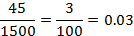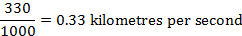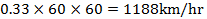# Conversions

First let’s take a look at some simple conversions.

1kg=1000grams

1litre=1000ml

1metre=100centimetres

1km=1000metres=100000centimetres

1minute=60seconds

1hour=60minute=3600seconds

1.1

Express 45 centimetres as a fraction of 15 metres and write your answer in its simplest form.

Express everything in centimetres

15metres= 1500centimetres1.2

Given that the speed of sound in air is 330 metres per second, express this speed in km/hr

Change metres to kilometres and then seconds to hours1.3

Express 320 ...